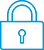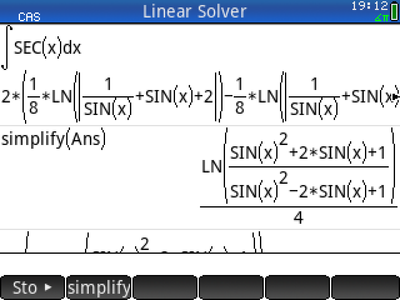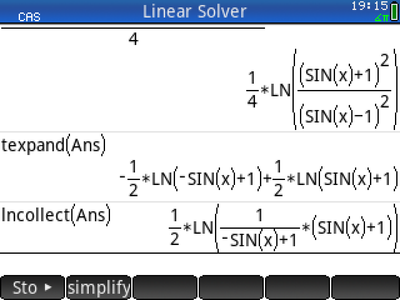cancel
Showing results for
Did you mean:It has been a while since anyone has replied. Simply ask a new question if you would like to start the discussion again.New member
1 0 0 0
Message 1 of 2
1,237
Flag Post

Solved!

# Integrate of sec(x)HP Recommended
HP prime

Hi, I'm learning how to use the HP Prime, and I've noticed that when I integrate Sec(x) it gives me a long answer instead of LnIsecx+tgxI, also when I calculate a determinate including a SEC(x) it gives me a long result . is there a way to make it appear Sec in the results?

Tags (1)
1 ACCEPTED SOLUTION

Accepted SolutionsLevel 6
305 297 32 76
Message 2 of 2
Flag PostHP Recommended

hello,

I do not know an easy way to accomplish  this conversion.

What I can do is this:By multiplying both the numerator and the denominator of the trigoniometric expression by (1+sin(x)), the numerator will become (1+sin(x))^2 and the denominator (cos(x))^2

By taking its root and replacing the 1/2 in front by 1, the trigoniometric expression will become

(1+sin(x))/cos(x)=sec(x)+tan(x), which is the simple result.

Tags (1)Level 6
305 297 32 76
Message 2 of 2
Flag PostHP Recommended

hello,

I do not know an easy way to accomplish  this conversion.

What I can do is this:By multiplying both the numerator and the denominator of the trigoniometric expression by (1+sin(x)), the numerator will become (1+sin(x))^2 and the denominator (cos(x))^2

By taking its root and replacing the 1/2 in front by 1, the trigoniometric expression will become

(1+sin(x))/cos(x)=sec(x)+tan(x), which is the simple result.

Tags (1)
† The opinions expressed above are the personal opinions of the authors, not of HP. By using this site, you accept the Terms of Use and Rules of Participation